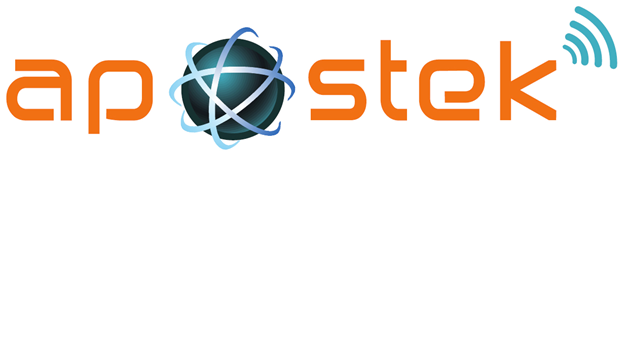Easy Queries
Tag(s):

## Advanced Data Structures, Data Structures, Easy, Segment Trees

Problem
Editorial
Analytics

You are given an array of size $N$ in which the value of the elements are either $0$ or $1$. All the elements of array are numbered from position $0$ to $N-1$.You are given some queries which can be of following $2$ types.

$0 \; index$ : In this type of query you have to find the nearest left and nearest right element from position $index$ in the array whose value is $1$.
$1 \; index$ : In this type of query you need to change the value at positon $index$ to $1$ if its previous value is 0 or else leave it unchanged.

Important Note : If there is no position with value 1 on the left side of any index in query then print -1 instead of left index and similarly if there is no value 1 in right side of the value index in that query then print -1 in place of the answer for right element.

Input
First line contains $2$ integers $N$ and $Q$ denoting the number of elements in array and number of queries.
Next line contains $N$ elements denoting the array.
Next $Q$ lines contains $2$ integer each denoting the any type of Query.

Output
For each query print left and right nearest element seprated by space.

Constraints
$1 \le N \le 100000$
$1\le index \le N$

Sample Inputs

 Input Output 5 4 0 0 0 0 0 0 2 1 2 1 4 0 3 -1 -1 2 4 6 2 1 0 1 0 1 1 0 0 0 4 -1 2 2 5
SAMPLE INPUT
7 4
1 0 0 0 1 0 1
0 1
0 5
1 2
0 2
SAMPLE OUTPUT
0 4
4 6
0 4
Explanation

The First query is 0 1. So the nearest 1 in left side from index 1 is element at index 0 and in right side the element is at index 4.

Similarly, the second query is 0 5. So the nearest 1 in left side from index 5 is element at index 4 and in right side the element is at index 6.

The third query is 1 2. So set the value equal to 1 of the element at index 2.

The Fourth query is 0 2. So the nearest 1 in left side from index 1 is element at index 2 and in right side the element is at index 4.

Time Limit: 1.0 sec(s) for each input file.
Memory Limit: 256 MB
Source Limit: 1024 KB
Marking Scheme: Marks are awarded when all the testcases pass.
Allowed Languages: Bash, C, C++, C++14, Clojure, C#, D, Erlang, F#, Go, Groovy, Haskell, Java, Java 8, JavaScript(Rhino), JavaScript(Node.js), Julia, Kotlin, Lisp, Lisp (SBCL), Lua, Objective-C, OCaml, Octave, Pascal, Perl, PHP, Python, Python 3, R(RScript), Racket, Ruby, Rust, Scala, Swift, Swift-4.1, TypeScript, Visual Basic

## CODE EDITOR

Initializing Code Editor...
Your Rating:

## This Problem was Asked inChallenge Name

Apostek Java Hiring Challenge

OTHER PROBLEMS OF THIS CHALLENGE
• Basic Programming > Input/Output
• Data Structures > Trees

?